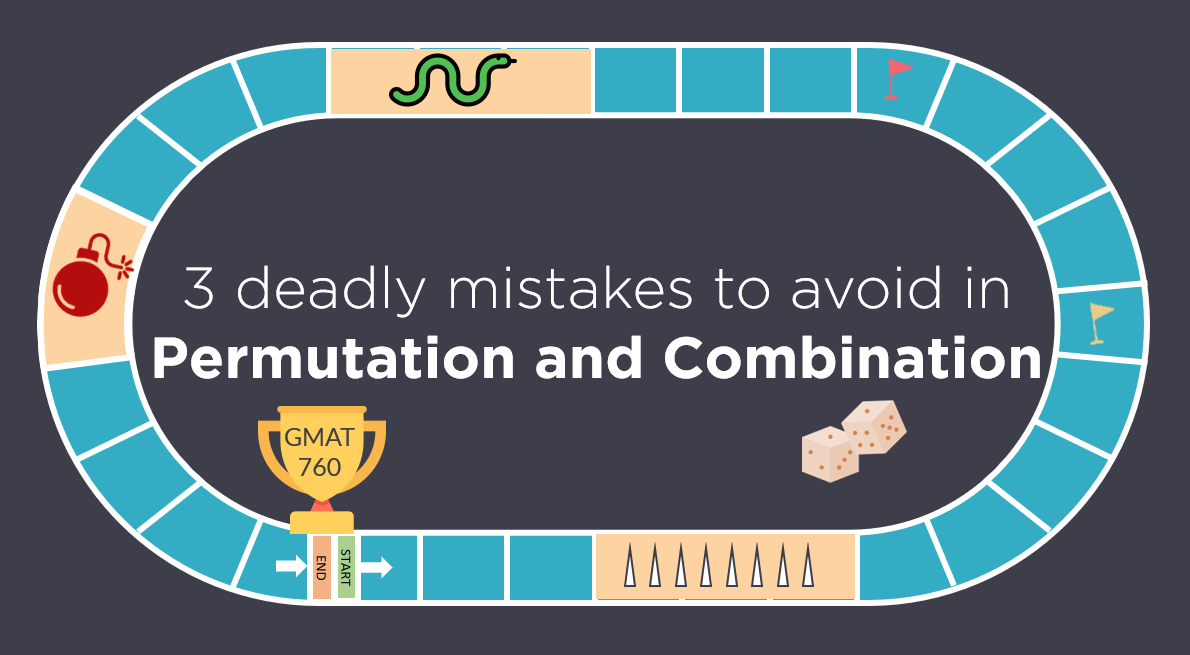# Permutation and Combination | Avoid these 3 Mistakes | GMAT Quant

Like any GMAT Quant topic, Permutation and Combination has its own traps. Most students fall in these traps and ultimately, are not able to secure their target GMAT score. In this article, we will highlight 3 most common mistakes that students make in Permutation and Combination problems along with the ways to avoid the mistake. We

Read More# Difference between Permutation and Combination explained with examples

Permutations and Combinations is a topic full of conundrums. The biggest one is, understanding the difference between permutation and combination. Should I solve this question using permutation or combination? In this article, we will give you a foolproof method to differentiate between the two. In the last article of ‘Permutation and Combination’ series we talked

Read More# How to use Tree Structures to solve GMAT arithmetic problems

Read More# P is a particle on the circle shown above. What is the length of the path travelled by P in one complete revolution around the circle? – OGQR 2020 Question #212 with Solution

[two_third] OGQR 2020: Question No. 212 P is a particle on the circle shown above. What is the length of the path travelled by P in one complete revolution around the circle? The diameter of the circle is 1.5 meters. The particle P moves in a clockwise direction at 0.5 meter per second. [/two_third] [one_third_last] Source OGQR 2020 Type Data Sufficiency Topic

Read More# What was the population of City X in 2002? – OGQR 2020 Question #209 with Solution

[two_third] OGQR 2020: Question No. 209 What was the population of City X in 2002? X’s population in 2002 increased by 2 percent, or 20,000 people, over 2001. In 2001, X’s population was 1,000,000. [/two_third] [one_third_last] Source OGQR 2020 Type Data Sufficiency Topic Word Problems Sub-Topic Percentages Difficulty Medium [/one_third_last] Solution Steps 1 & 2: Understand Question

Read More# Carl averaged 2m miles per hour on a trip that took him h hours. If Ruth made the same trip in h hours, what was her average speed in miles per hour? – OGQR 2020 Question #82 with Solution

[two_third] OGQR 2020: Question No. 82 Carl averaged 2m miles per hour on a trip that took him h hours. If Ruth made the same trip in (2/3)h hours, what was her average speed in miles per hour? [/two_third] [one_third_last] Source OGQR 2020 Type Problem Solving Topic Word Problems Sub-Topic Distance Difficulty Easy-Medium [/one_third_last] Solution

Read More# If Car A took n hours to travel 2 miles and Car B took m hours to travel 3 miles, which of the following expresses the time it would take Car C, traveling at the average (arithmetic mean) of those rates, to travel 5 miles? – OGQR 2020 Question #79 with Solution

[two_third] OGQR 2020: Question No. 79 If Car A took n hours to travel 2 miles and Car B took m hours to travel 3 miles, which of the following expresses the time it would take Car C, traveling at the average (arithmetic mean) of those rates, to travel 5 miles? [/two_third] [one_third_last] Source OGQR

Read More# A paint mixture was formed by mixing exactly 3 colors of paint. By volume, the mixture was x% blue paint, y% green paint, and z% red paint. If exactly 1 gallon of blue paint and 3 gallons of red paint were used, how many gallons of green paint were used? – OG 2020 Question #346 with Solution

[two_third] OG 2020: Question No. 346 A paint mixture was formed by mixing exactly 3 colors of paint. By volume, the mixture was x% blue paint, y% green paint, and z% red paint. If exactly 1 gallon of blue paint and 3 gallons of red paint were used, how many gallons of green paint were

Read More# A company produces a certain toy in only 2 sizes, small or large, and in only 2 colors, red or green. If, for each size, there are equal numbers of red and green toys in a certain production lot, what fraction of the total number of green toys is large? – OG 2020 Question #323 with Solution

Read MoreRead More